Hi, I was wondering if you could explain to me how x + y= π/2, sin x = cos y. Thank you!

To understand this rule (which obviously works in degrees just as it does in radians), turn to right triangles.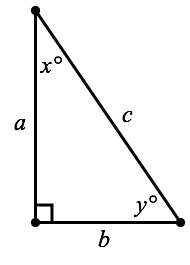Below, you’ve got a right triangle with acute angles measuring x° and y°. Because the third angle in the triangle is a right angle, and because the angles in any triangle add up to 180°, we know that no matter what x and y are,
= 90°.

So far so good?

Now let’s list out some trigonometric ratios using out old pal SOH-CAH-TOA (well, really just the SOH and CAH parts for this demonstration).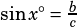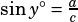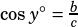There you have it.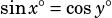and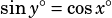, just by nature of x° and y° being the measures of the two acute angles in the same right triangle.

The trick is, for any two angles that add up to 90°, you can draw a right triangle that contains them. Therefore, for any two angles that add up to 90° (or π/2 radians) x and y, sin x = cos y and sin y = cos x.